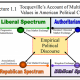# Nonparametric Statistical Analysis Using R: The Sign Test

Using R in Nonparametic Statistical Analysis:  The Binomial Sign Test

A tutorial by D.M. Wiig

One of the core competencies that students master in introductory social science statistics is to create a null and alternative hypothesis pair relative to a research question and to use a statistical test to evaluate and make a decision about rejecting or retaining the null hypothesis.  I have found that one of the easiest statistical tests to use when teaching these concepts is the sign test.  This is a very easy test to use and students seem to intuitively grasp the concepts of trials and binomial outcomes as these are easily related to the common and familiar event of ‘flipping a coin.’

While it is possible to use the sign test by looking up probabilities of outcomes in a table of the binomial distribution I have found that using R to perform the analysis is a good way to get them involved in using statistics software to solve the problem.  R has an easy to use sign test routine that is called with the binom.test command.  To illustrate the use of the test consider an experiment where the researcher has randomly assigned 10 individuals to a group and observes them in both a control and experimental condition.  The researcher measures the criterion variable of interest in each condition for each subject and measures the effect on each subject’s behavior using a relative scale of effect.

The researcher at this point is only interested in whether or not the criterion variable has an effect on behavior, so a non-directional hypothesis is used.  The data collected is shown in the following table:

Subject   1     2     3     4     5     6      7      8     9     10

———————————————————————-

Pre      50   49   37   16   80   42    40    58   31    21

Post.   56   50   30   25   90   44    60    71   32    22

———————————————————————–

+     +     –     +     +     +      +      +    +      –

The general format for the sign test is as follows:

binom.test(x, n, p =.5, alternative = “two.sided”, “less”, “greater”, conf.level = .95)

where: x = number of successes

n = number of trials

alternative = indicates the alternative hypthesis as directional or nondirectional

conf.level = the confidence level for the returned confidence interval.

In the example as described above we have 8 pluses and 2 minuses.  We will use the “two.sided” option for the alternative hypothesis a probability of success of .50, and a conf.level of .95. The following is entered into R:

Under a nondirectional alternative hypothesis we are testing the probability of obtaining 0, 1, 2, 8, 9, 10 pluses or:

> binom.test(8, 10, p=.5, alternative=”two.sided”, conf.level=.95)

Exact binomial test

data:  8 and 10
number of successes = 8, number of trials = 10,
p-value = 0.1094
alternative hypothesis: true probability of success is not equal to 0.5
95 percent confidence interval:
0.4439045 0.9747893
sample estimates:
probability of success
0.8
 >

Under a nondirectional alternative hypothesis we are testing the probability of obtaining 0, 1, 2, 8, 9, 10 pluses or:

p(o, 1, 2, 8, 9, 10 pluses)  = .1094

If we had set an alpha of ά=.05 then we would retain the null hypothesis as p(obt) > .05.  We could not conclude that the experimental criterion has an effect on behavior.  R has many other nonparametric statistical tests that are easy to use from the command line.  These are topics for future tutorials.

More to Follow:

## One thought on “Nonparametric Statistical Analysis Using R: The Sign Test”

1.Political Pipeline says:

Reblogged this on Political Pipeline and commented:
rejecting the null is useful, yet is the social sciences we may transition to Bayesian approach considering the data as a “prior” and then, as data analysts, we track and later redo the analysis (posterior), which helps us observe change and, indubitably, trajectory.

Like

This site uses Akismet to reduce spam. Learn how your comment data is processed.